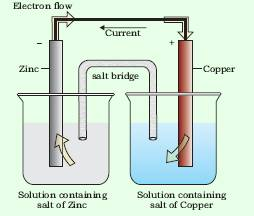Chapter 8 Redox Reactions

 Oxidation Reduction 1.  Addition of oxygen 1.  Removal of oxygen 2.  Removal of hydrogen 2.  Addition of hydrogen 3.  Addition of an electronegative element 3.  Removal of an electronegative element 4.  Removal of an electropositive element 4.  Addition of an electropositive element 5.  Loss of electron 5.  Gain of electron

Oxidation number denotes theoxidation state of an element in a compound ascertained according to a setof rules formulated on the basis thatelectron in a covalent bond belongsentirely to more electronegative element.

### Calculation of oxidation number

1.  O. S. of all the elements in their elemental form (in standard state) is taken as zero O. S. of elements in Cl2, F2, O2, P4, O3, Fe(s), H2, N2, C(graphite) is zero.

2.   Common O. S. of elements of group one (1st) is one. Common O. S. of elements of group two (2nd) is two.

3.  For ions composed of only one atom, theoxidation number is equal to the chargeon the ion.

4.  The oxidation number of oxygen in most compounds is –2.While in peroxides (e.g., H2O2, Na2O2), eachoxygen atom is assigned an oxidationnumber of –1, in superoxides (e.g., KO,RbO2) each oxygen atom is assigned anoxidation number of –(½).

5.  In oxygendifluoride (OF2) and dioxygendifluoride (O2F2), the oxygen is assignedan oxidation number of +2 and +1,respectively.

6.  The oxidation number of hydrogen is +1 but in metal hydride its oxidation no. is–1.

7.  In all its compounds, fluorine has anoxidation number of –1.

8.  The algebraic sum of the oxidation numberof all the atoms in a compound must bezero.

9.  In polyatomic ion, the algebraic sumof all the oxidation numbers of atoms ofthe ion must equal the charge on the ion.
Stockotation:the oxidationnumber is expressed by putting a Romannumeral representing the oxidation numberin parenthesis after the symbol of the metal inthe molecular formula. Thus aurous chlorideand auric chloride are written as Au(I)Cl andAu(III)Cl3. Similarly, stannous chloride andstannic chloride are written as Sn(II)Cl2andSn(IV)Cl4.

Oxidation: An increase in the oxidationnumber
Reduction: A decrease in the oxidationnumber

Oxidising agent: A reagent which canincrease the oxidation number of an elementin a given substance. These reagents are calledas oxidants also.

Reducing agent: A reagent which lowers the oxidation number of an element in a givensubstance. These reagents are also called asreductants.

Redox reactions: Reactions which involvechange in oxidation number of the interactingspecies

#### Oxidation Number Method:

Write the net ionic equation for the reaction of potassium dichromate(VI), K2Cr2O7 with sodium sulphite,Na2SO3, in an acid solution to give chromium(III) ion and the sulphate ion.

Step 1: The skeletal ionic equation is: Cr2O72–(aq) + SO32–(aq) → Cr3+(aq)+ SO42–(aq)

Step 2: Assign oxidation numbers forCr andS+6 –2 +4 –2 +3 +6 –2 Cr2O72–(aq) + SO32–(aq) → Cr3+(aq)+ SO42–(aq)

Step 3: Calculate the increase anddecrease of oxidation number, and make them equal:+6 –2    +4 –2   +3   +6 Cr2O72–(aq) + 3SO32–(aq) → 2Cr3+(aq)+ 3SO42–(aq)

Step 4: Balance the charge by adding H+as the reaction occurs in theacidic medium, Cr2O72–(aq) + 3SO32-(aq) 8H+ → 2Cr3+(aq)+ 3SO42–(aq)

Step 5: Balance the oxygen atom by adding water molecule. Cr2O72–(aq) + 3SO32–(aq) 8H+→ 2Cr3+(aq)+ 3SO42–(aq)+ 4H2O(l)

#### Half Reaction Method

balance the equation showing the oxidation of Fe2.+ ions to Feb3+ ions by dichromate ions (Cr2O72– in acidic medium,wherein, Cr2 O72– ions are reduced to Cr3+ ions.

Step 1: Produce unbalanced equation for thereaction in ionic form: Fe2+(aq) + Cr2O72– (aq) → Fe3+ (aq) + Cr3+(aq)

Step 2: Separate the equation into halfreactions: +2 +3 Oxidation half : Fe2+ (aq) → Fe3+(aq) +6 –2 +3 Reduction half :Cr2O72–(aq) → Cr3+(aq)

Step 3: Balance the atoms other than O andH in each half reaction individually. Cr2O72– (aq) → Cr3+(aq)

Step 4: For reactions occurring in acidicmedium, add H2O to balance O atoms and H+to balance H atoms.Cr2O72– (aq) +14 H+→ Cr3+(aq) + 7H2O (l)

Step 5: Add electrons to one side of the halfreaction to balance the charges. If need be,make the number of electrons equal in the twohalf reactions by multiplying one or both halfreactions by appropriate coefficients. Fe2+ (aq) → Fe3+ (aq) + e– Cr2O72– (aq) + 14H+ (aq) + 6e– → 2Cr3+(aq) +7H2O (l) 6Fe2+ (aq) →6 Fe3+ (aq) +6 e–

Step 6: We add the two half reactions toachieve the overall reaction and cancel theelectrons on each side. This gives the net ionicequation as : 6Fe2+(aq) + Cr2O72– (aq) + 14H+(aq) → 6 Fe3+(aq) +2Cr 3+(aq) + 7H2O(l)

A redox couple is defined as havingtogether the oxidised and reduced forms of asubstance taking part in an oxidation orreduction half reaction. Represented as Zn2+/Zn and Cu2+/Cu.
v Electrochemical cells are the devices which are used to get electric current by using chemical reaction.

#### Electrochemical cells

Electrochemical cells are the devices which are used to get electric current by using chemical reaction.The potential associated with eachelectrode is known as electrode potential. Ifthe concentration of each species taking partin the electrode reaction is unity (if any gasappears in the electrode reaction, it is confinedto 1 atmospheric pressure) and further thereaction is carried out at 298K, then thepotential of each electrode is said to be the Standard Electrode Potential

•  SHE is used to measure electrode potential and its standard electrode potential is taken as 0.00 V.

### NCERT Books Free Pdf Download for Class 5, 6, 7, 8, 9, 10 , 11, 12 Hindi and English Medium

 Mathematics Biology Psychology Chemistry English Economics Sociology Hindi Business Studies Geography Science Political Science Statistics Physics Accountancy